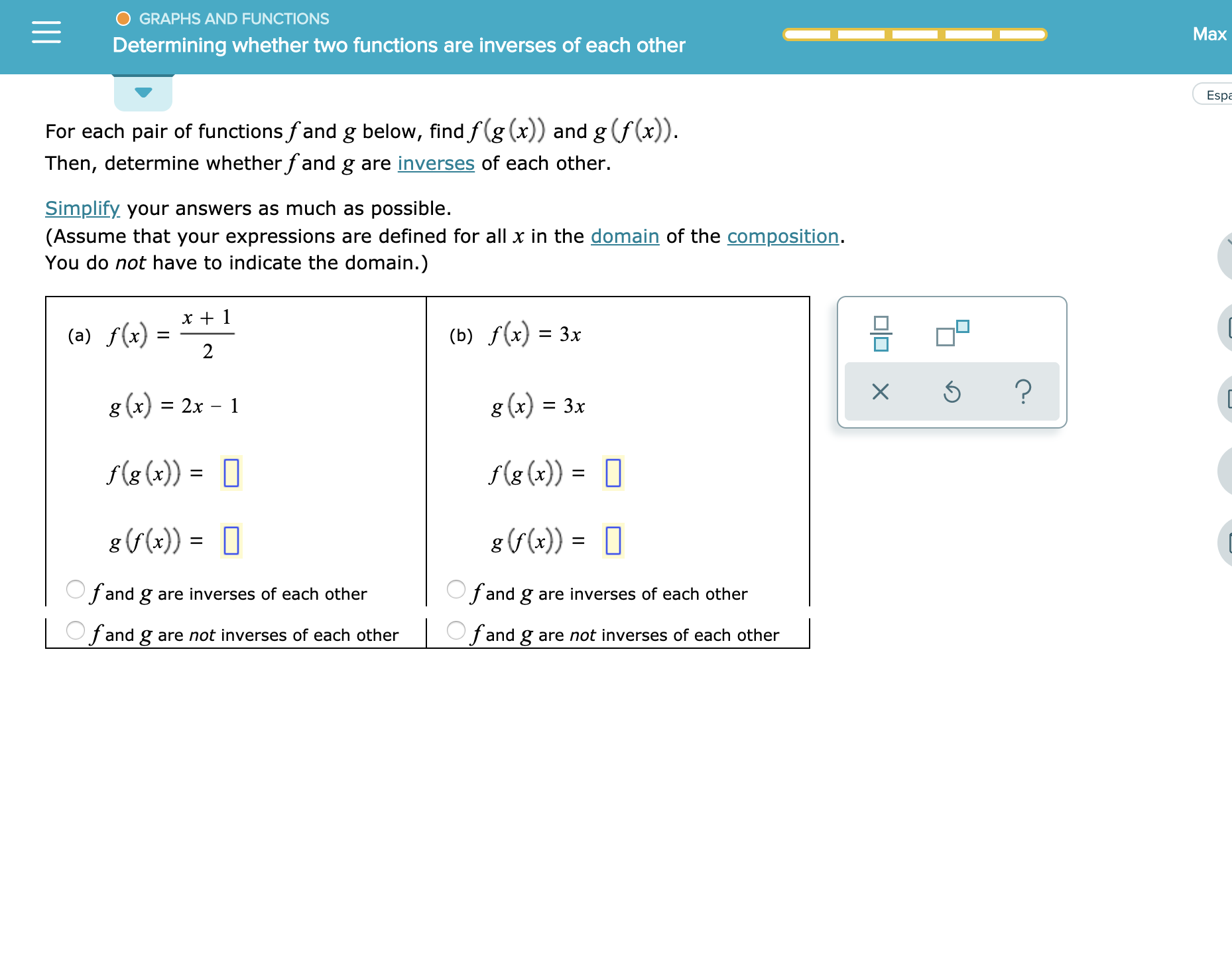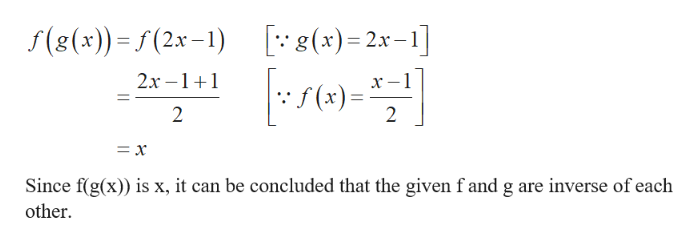# O GRAPHS AND FUNCTIONSMaxDetermining whether two functions are inverses of each otherEspaFor each pair of functions fand g below, find f(g (x)) and g f(x))Then, determine whether fand g are inverses of each other.Simplify your answers as much as possible(Assume that your expressions are defined for all x in the domain of the composition.You do not have to indicate the domain.)x 1(b) f(x) = 3x(a) f(x)2?g (x) 3xg (x) 2x - 1f(g (x))f(g(x))g (r())=fand g are inverses of each otherfand g are inverses of each otherOfand gare not inverses of each otherfand g are not inverses of each otherX

Question
2 views

See attachmenthelp_outlineImage TranscriptioncloseO GRAPHS AND FUNCTIONS Max Determining whether two functions are inverses of each other Espa For each pair of functions fand g below, find f(g (x)) and g f(x)) Then, determine whether fand g are inverses of each other. Simplify your answers as much as possible (Assume that your expressions are defined for all x in the domain of the composition. You do not have to indicate the domain.) x 1 (b) f(x) = 3x (a) f(x) 2 ? g (x) 3x g (x) 2x - 1 f(g (x)) f(g(x)) g (r()) = fand g are inverses of each other fand g are inverses of each other Ofand gare not inverses of each other fand g are not inverses of each other X fullscreen
check_circle

Step 1

Calculation:

a)

Compute f(g(x)) a...help_outlineImage Transcriptionclosef(8(x)) f(2x-1) (x)2x-] - 2х-1 _ 2х -1+1 |5 (x) = 2 2 = x Since f(g(x)) is x, it can be concluded that the given f and g are inverse of each other fullscreen

### Want to see the full answer?

See Solution

#### Want to see this answer and more?

Solutions are written by subject experts who are available 24/7. Questions are typically answered within 1 hour.*

See Solution
*Response times may vary by subject and question.
Tagged in

### Other